• Slides: 11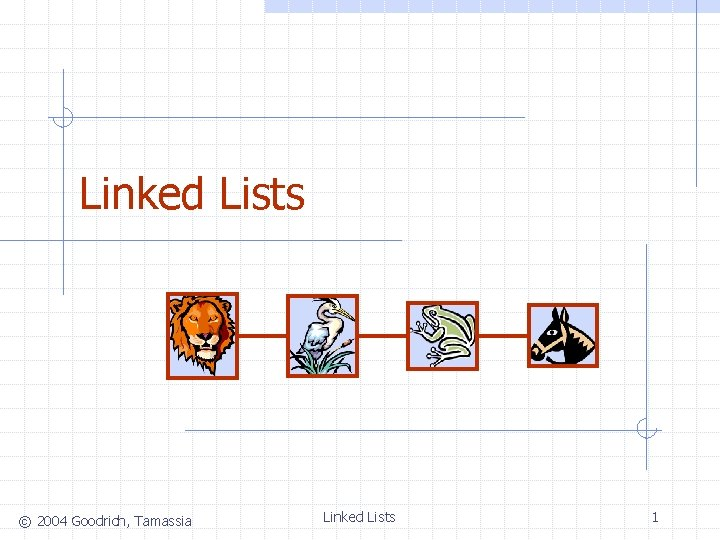Singly Linked List (§ 4. 4. 1) A singly linked list is a concrete data structure consisting of a sequence of nodes Each node stores n n next element link to the next node elem A © 2004 Goodrich, Tamassia B C Linked Lists D 2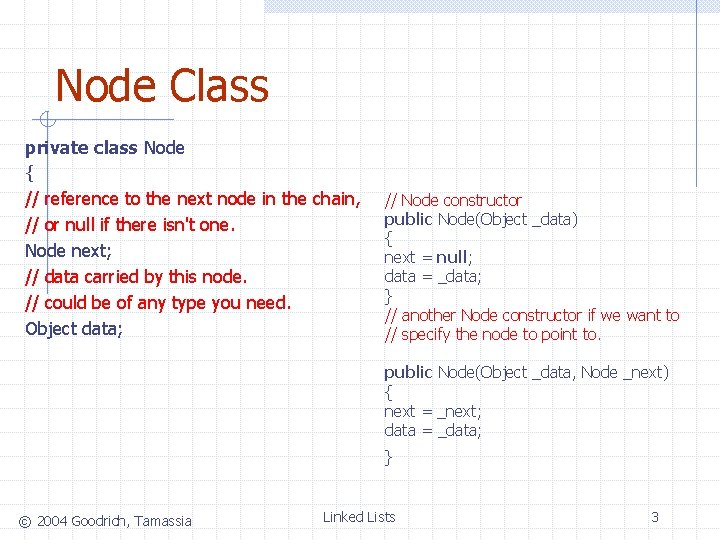Node Class private class Node { // reference to the next node in the chain, // or null if there isn't one. Node next; // data carried by this node. // could be of any type you need. Object data; // Node constructor public Node(Object _data) { next = null; data = _data; } // another Node constructor if we want to // specify the node to point to. public Node(Object _data, Node _next) { next = _next; data = _data; } © 2004 Goodrich, Tamassia Linked Lists 3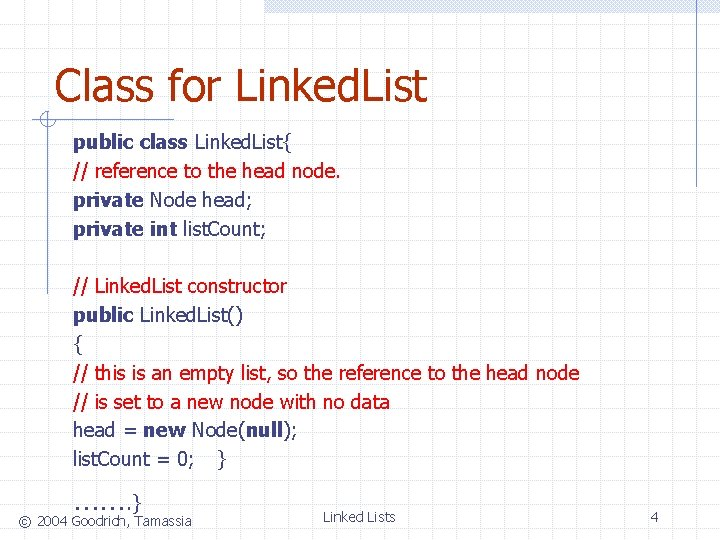Class for Linked. List public class Linked. List{ // reference to the head node. private Node head; private int list. Count; // Linked. List constructor public Linked. List() { // this is an empty list, so the reference to the head node // is set to a new node with no data head = new Node(null); list. Count = 0; } ……. } © 2004 Goodrich, Tamassia Linked Lists 4public void add(Object data) // post: appends the specified element to the end of this list. { Node temp = new Node(data); Node current = head; // starting at the head node, crawl to the end of the list while(current. get. Next() != null) { current = current. get. Next(); } // the last node's "next" reference set to our new node current. set. Next(temp); list. Count++; // increment the number of elements variable } © 2004 Goodrich, Tamassia Linked Lists 5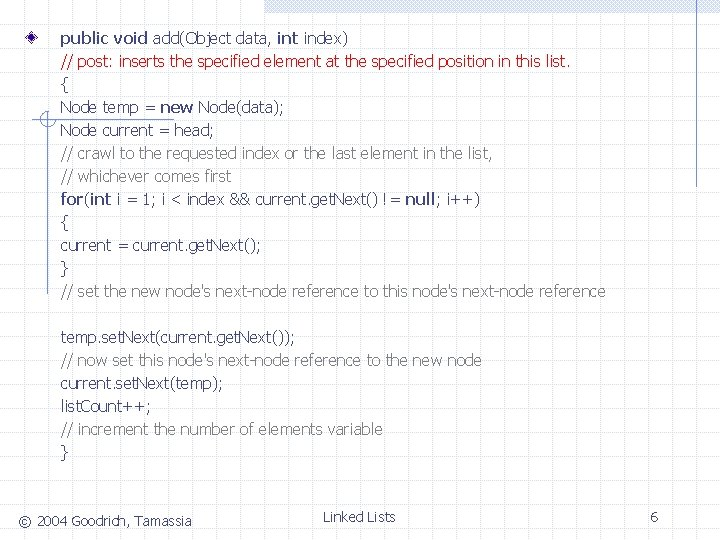public void add(Object data, int index) // post: inserts the specified element at the specified position in this list. { Node temp = new Node(data); Node current = head; // crawl to the requested index or the last element in the list, // whichever comes first for(int i = 1; i < index && current. get. Next() != null; i++) { current = current. get. Next(); } // set the new node's next-node reference to this node's next-node reference temp. set. Next(current. get. Next()); // now set this node's next-node reference to the new node current. set. Next(temp); list. Count++; // increment the number of elements variable } © 2004 Goodrich, Tamassia Linked Lists 6public Object get(int index) // post: returns the element at the specified position in this list. { // index must be 1 or higher if(index <= 0) return null; Node current = head. get. Next(); for(int i = 1; i < index; i++) { if(current. get. Next() == null) return null; current = current. get. Next(); } return current. get. Data(); } © 2004 Goodrich, Tamassia Linked Lists 7public boolean remove(int index) // post: removes the element at the specified position in this list. { // if the index is out of range, exit if(index < 1 || index > size()) return false; Node current = head; for(int i = 1; i < index; i++) { if(current. get. Next() == null) return false; current = current. get. Next(); } current. set. Next(current. get. Next()); list. Count--; // decrement the number of elements variable return true; } © 2004 Goodrich, Tamassia Linked Lists 8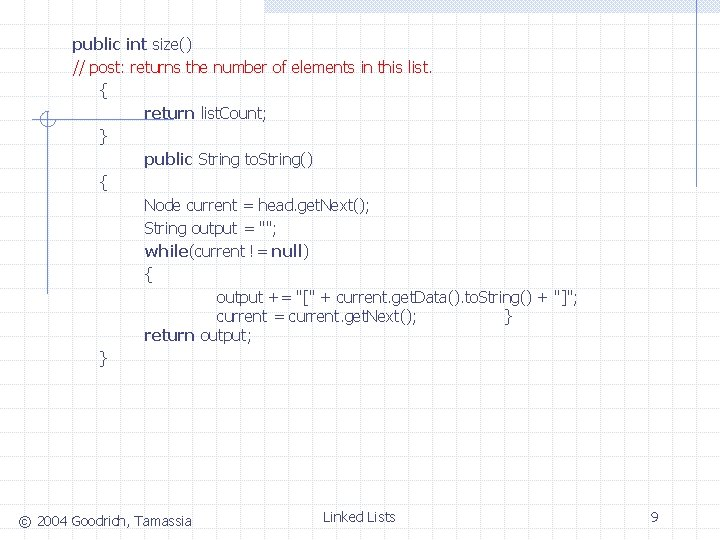public int size() // post: returns the number of elements in this list. { return list. Count; } public String to. String() { Node current = head. get. Next(); String output = ""; while(current != null) { output += "[" + current. get. Data(). to. String() + "]"; current = current. get. Next(); } return output; } © 2004 Goodrich, Tamassia Linked Lists 9// these methods should be self-explanatory public Object get. Data() { return data; } public void set. Data(Object _data) { data = _data; } public Node get. Next() { return next; } public void set. Next(Node _next) { next = _next; } © 2004 Goodrich, Tamassia Linked Lists 10